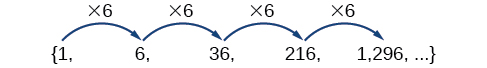# 13.3 Geometric sequences

 Page 1 / 6
In this section, you will:
• Find the common ratio for a geometric sequence.
• List the terms of a geometric sequence.
• Use a recursive formula for a geometric sequence.
• Use an explicit formula for a geometric sequence.

Many jobs offer an annual cost-of-living increase to keep salaries consistent with inflation. Suppose, for example, a recent college graduate finds a position as a sales manager earning an annual salary of $26,000. He is promised a 2% cost of living increase each year. His annual salary in any given year can be found by multiplying his salary from the previous year by 102%. His salary will be$26,520 after one year; $27,050.40 after two years;$27,591.41 after three years; and so on. When a salary increases by a constant rate each year, the salary grows by a constant factor. In this section, we will review sequences that grow in this way.

## Finding common ratios

The yearly salary values described form a geometric sequence because they change by a constant factor each year. Each term of a geometric sequence increases or decreases by a constant factor called the common ratio . The sequence below is an example of a geometric sequence because each term increases by a constant factor of 6. Multiplying any term of the sequence by the common ratio 6 generates the subsequent term.## Definition of a geometric sequence

A geometric sequence    is one in which any term divided by the previous term is a constant. This constant is called the common ratio    of the sequence. The common ratio can be found by dividing any term in the sequence by the previous term. If ${a}_{1}$ is the initial term of a geometric sequence and $r$ is the common ratio, the sequence will be

Given a set of numbers, determine if they represent a geometric sequence.

1. Divide each term by the previous term.
2. Compare the quotients. If they are the same, a common ratio exists and the sequence is geometric.

## Finding common ratios

Is the sequence geometric? If so, find the common ratio.

1. $1\text{,}\text{\hspace{0.17em}}2\text{,}\text{\hspace{0.17em}}4\text{,}\text{\hspace{0.17em}}8\text{,}\text{\hspace{0.17em}}16\text{,}\text{\hspace{0.17em}}...$

Divide each term by the previous term to determine whether a common ratio exists.

1. $\begin{array}{llllllllll}\frac{2}{1}=2\hfill & \hfill & \hfill & \frac{4}{2}=2\hfill & \hfill & \hfill & \frac{8}{4}=2\hfill & \hfill & \hfill & \frac{16}{8}=2\hfill \end{array}$

The sequence is geometric because there is a common ratio. The common ratio is 2.

2. $\begin{array}{lllllll}\frac{12}{48}=\frac{1}{4}\hfill & \hfill & \hfill & \frac{4}{12}=\frac{1}{3}\hfill & \hfill & \hfill & \frac{2}{4}=\frac{1}{2}\hfill \end{array}$

The sequence is not geometric because there is not a common ratio.

If you are told that a sequence is geometric, do you have to divide every term by the previous term to find the common ratio?

No. If you know that the sequence is geometric, you can choose any one term in the sequence and divide it by the previous term to find the common ratio.

Is the sequence geometric? If so, find the common ratio.

$5,10,15,20,...$

The sequence is not geometric because $\frac{10}{5}\ne \frac{15}{10}$ .

Is the sequence geometric? If so, find the common ratio.

$100,20,4,\frac{4}{5},...$

The sequence is geometric. The common ratio is $\frac{1}{5}$ .

## Writing terms of geometric sequences

Now that we can identify a geometric sequence, we will learn how to find the terms of a geometric sequence if we are given the first term and the common ratio. The terms of a geometric sequence can be found by beginning with the first term and multiplying by the common ratio repeatedly. For instance, if the first term of a geometric sequence is ${a}_{1}=-2$ and the common ratio is $r=4,$ we can find subsequent terms by multiplying $-2\cdot 4$ to get $-8$ then multiplying the result $-8\cdot 4$ to get $-32$ and so on.

Find the possible value of 8.5 using moivre's theorem
which of these functions is not uniformly cintinuous on (0, 1)? sinx
which of these functions is not uniformly continuous on 0,1
solve this equation by completing the square 3x-4x-7=0
X=7
Muustapha
=7
mantu
x=7
mantu
3x-4x-7=0 -x=7 x=-7
Kr
x=-7
mantu
9x-16x-49=0 -7x=49 -x=7 x=7
mantu
what's the formula
Modress
-x=7
Modress
new member
siame
what is trigonometry
deals with circles, angles, and triangles. Usually in the form of Soh cah toa or sine, cosine, and tangent
Thomas
solve for me this equational y=2-x
what are you solving for
Alex
solve x
Rubben
you would move everything to the other side leaving x by itself. subtract 2 and divide -1.
Nikki
then I got x=-2
Rubben
it will b -y+2=x
Alex
goodness. I'm sorry. I will let Alex take the wheel.
Nikki
ouky thanks braa
Rubben
I think he drive me safe
Rubben
how to get 8 trigonometric function of tanA=0.5, given SinA=5/13? Can you help me?m
More example of algebra and trigo
What is Indices
If one side only of a triangle is given is it possible to solve for the unkown two sides?
cool
Rubben
kya
Khushnama
please I need help in maths
Okey tell me, what's your problem is?
Navin
the least possible degree ?
(1+cosA)(1-cosA)=sin^2A
good
Neha
why I'm sending you solved question
Mirza
Teach me abt the echelon method
Khamis
exact value of cos(π/3-π/4)
What is differentiation?ByByByBy